# SQL Server ML Services - Multiple Input Data Sets

Posted by nielsb on Monday, December 24, 2018

This post came about due to a question on the Microsoft Machine Learning Server forum. The question was if there are any plans by Microsoft to support more the one input dataset (`@input_data_1`) in `sp_execute_external_script`. My immediate reaction was that if you want more than one dataset, you can always connect from the script back into the database, and retrieve data.

However, the poster was well aware of that, but due to certain reasons he did not want to do it that way - he wanted to push in the data, fair enough. When I read this, I seemed to remember something from a while ago, where, instead of retrieving data from inside the script, they pushed in the data, serialized it as an output parameter and then used the binary representation as in input parameter (yeah - this sounds confusing, but bear with me). I did some research (read Googling), and found this StackOverflow question, and answer. So for future questions, and for me to remember, I decided to write a blog post about it.

DISCLAIMER: I want to make it perfectly clear that the method outlined in this post is NOT my idea, but - as mentioned above - comes from the StackOverflow answer by Brandon Moretz.

## Recap

We start with a recap about how we pass/retrieve data for external scripts. In Microsoft SQL Server R Services - sp_execute_external_script - I we discussed, among other things, the `sp_execute_external_script`’s `@input_data_1` parameter and how it specifies the input data used by the external script in the form of a Transact-SQL query:

 ``````1 2 3 4 5 6 7 8 `````` ``````EXEC sp_execute_external_script @language = N'R', @script = N' iris_dataset <- InputDataSet setosa <- iris_dataset[iris_dataset\$Species == "setosa",] meanSepWidth <- mean(setosa\$SepalWidth) cat(paste("Seposa sepal mean width: ", meanSepWidth))', @input_data_1 = N'SELECT * FROM dbo.tb_irisdata_full' ``````

Code Snippet 1: Using @input_data_1 with straight SELECT

In Code Snippet 1 we see how the `@input_data_1` parameter contains a `SELECT` statement against a table, and the statement executes during the `sp_execute_external_script` execution. The dataset generated by the query is referred to in the script as `InputDataSet`. The query has to be based on a `SELECT`, but it does not have to be against a table, it can be against a view or a user-defined function as well.

NOTE: The code in Code Snippet 1 above, and Code Snippet 2 below, uses tables and data from the Microsoft SQL Server R Services - sp_execute_external_script - I post.

That is all well and good, but what if you want to push in multiple datasets? Seeing that the parameter we use to define the data ends with a `1`, it would be logical to think there are `@input_data_2`, `3`, and so on - but no, that is not the case. To process more than one dataset in the external script, we have to pull the dataset(s) from inside the script. To do this, we can use `ODBC` (in R it is the `RODBC` package, in Python `pyodbc`) or the highly optimized Microsoft `RevoScaleR` package (in Python `revoscalepy`). In the following example I use `RevoScaleR`:

 `````` 1 2 3 4 5 6 7 8 9 10 11 12 13 14 15 16 17 18 19 20 21 22 23 24 25 26 27 28 29 30 `````` ``````EXEC sp_execute_external_script @language = N'R', @script = N' # set up connection string sqlConnString <- "Driver=SQL Server;server=win10-dev; database=IrisTestDb;uid=;pwd=" # define the data mydata <- RxSqlServerData(table = NULL, sqlQuery = "SELECT * FROM dbo.tb_irisdata_uneven", connectionString = sqlConnString) # open the dataset rxOpen(mydata) # read the data iris_uneven <- rxReadNext(mydata) versicolor <- iris_uneven[iris_uneven\$Species == "versicolor",] meanSepWidthVersi <- mean(versicolor\$SepalWidth) # get the data from the input data set iris_dataset <- InputDataSet setosa <- iris_dataset[iris_dataset\$Species == "setosa",] meanSepWidth <- mean(setosa\$SepalWidth) # output the data cat(paste("Seposa sepal mean width:", meanSepWidth, ".", "Versicolor sepal mean width:", meanSepWidthVersi))', @input_data_1 = N'SELECT * FROM dbo.tb_irisdata_even'; ``````

Code Snippet 2: Multiple Data Sets using RevoScaleR

The code in Code Snippet 2 shows how we read in data within the script by the use of `RevoScaleR` functionality together with the data from the `@input_data_1` parameter, and how we subsequently calculate `mean` on the two datasets.

So above we see how we can use multiple datasets, but what if we for some or another do not want to / cannot connect from the script to the database, as per the question above? Well, that is what we cover in this post.

## Multiple Datasets

What we want to do is to push data from three completely different tables into the external script, and then in the script do something. We use `@input_data_1` to push one of the datasets, and we look at two different ways to push in the data from the other two tables. The tables we read data from are three system tables, this way we do not need to create separate tables and load data etc.:

• `sys.tables`.
• `sys.databases`.
• `sys.columns`.

What we want to do in the script is to use R/Python functionality to print out the number of rows that we push in from the separate tables, so we start with some code like this:

 ``````1 2 3 4 5 6 7 `````` ``````EXEC sp_execute_external_script @language = N'R' , @script = N' df <- InputDataSet nmbrRows <- nrow(df) cat(paste("Number rows @input_data_1: ", nmbrRows))' , @input_data_1 = N'SELECT * FROM sys.columns' ``````

Code Snippet 3: Pushing Data via @input_data_1 in R

There is nothing strange in Code Snippet 3, we see how we do the `SELECT` in `@input_data_1`, and how we in the script:

• Assign the `InputDataSet` to a variable: `df`.
• Use the R function `nrow` to get the number of rows in the data frame (`df`).
• Print it out to the console.

When we execute the code in Code Snippet 3, the result is like so: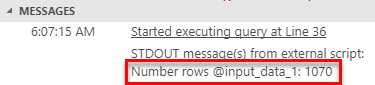Figure 1: Input Data 1 Result

From Figure 1 we see that the dataset contains 1070 rows.

If we want to do this in Python the code looks like this:

 ``````1 2 3 4 5 6 7 `````` ``````EXEC sp_execute_external_script @language = N'Python' , @script = N' df = InputDataSet nmbrRows = len(df.index) print("Number rows @input_data_1: ", nmbrRows)' , @input_data_1 = N'SELECT * FROM sys.columns' ``````

Code Snippet 4: Pushing Data via @input_data_1 in Python

Nothing strange in Code Snippet 4 either. The one noteworthy thing is the use of `len(df.index)`, instead of `count()` or `shape`. I use `len(df.index)` as “people in the know” says it performs better. When we execute the code in Code Snippet 4, we get the same result as we see in Figure 1.

Ok, so the code in the two code snippets above, (3 and 4), is the base code for what we want to do. Now we need to figure out how to push two more datasets into the scripts, and there are two ways to do that:

• JSON.
• Binary serialization.

Before we look at those two ways, let us discuss briefly a requirement for us to be able to do what we want, and that is the use of the `@params` parameter in `sp_execute_external_script`.

#### @params

The `@params` parameter is an optional parameter, and when defined it contains a comma separated list of the definitions of all parameters embedded in the values for the `@input_data_1` and the `@script` parameters. The string must be either a Unicode constant or a Unicode variable. Each parameter definition consists of a parameter name and a data type. The parameters defined in the `@params` list need to be added as named parameters to the stored procedure, and in the case of parameters for the external script; the script references the parameters by name but without the `@` sign.

So, when we push data into the script, either by using JSON or binary serialization we define a parameter for the data which we then reference in the script.

NOTE: If you want to get the inner workings of the `@params` parameter, have a look at my blog post: Microsoft SQL Server R Services - sp_execute_external_script - II.

Enough of this preamble, let us get going.

#### JSON

With the release of SQL Server 2016, Microsoft added support for JSON text processing. Microsoft added JSON functions to SQL Server, which enable you to analyze and query JSON data, transform JSON to relational format, and export SQL query results as JSON text. A typical query producing JSON text can look like this:

 ``````1 2 3 `````` ``````SELECT name, object_id, schema_id FROM sys.tables FOR JSON AUTO; ``````

Code Snippet 5: Retrieve JSON Data

We see how I use the `FOR JSON AUTO` syntax in Code Snippet 5 to indicate to SQL Server I want JSON formatted data as the resultset. You do not necessarily need to use `AUTO`, as there are other options. To see more of this look here: Format Query Results as JSON with FOR JSON (SQL Server).

I limit the columns I select to get a more readable output. When I execute and click on the result I see: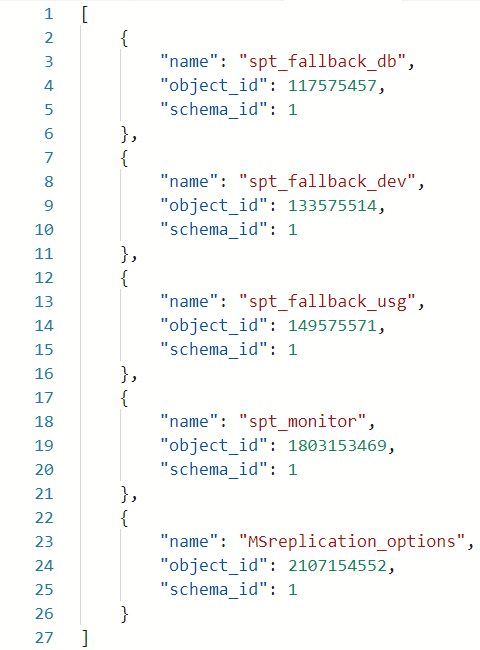Figure 2: JSON Result

So in Figure 2 we see how my `SELECT` query results in JSON data.

To solve our problem how to push in multiple datasets, we can now use the JSON formatted data together with the `@params` parameter to define two parameters containing JSON. For example, `@sysTables` and `@sysDatabases`:

 `````` 1 2 3 4 5 6 7 8 9 10 11 12 13 `````` ``````DECLARE @sysTabs nvarchar(max) = (SELECT * FROM sys.tables FOR JSON AUTO); DECLARE @sysDbs nvarchar(max) = (SELECT * FROM sys.databases FOR JSON AUTO); EXEC sp_execute_external_script @language = N'Python' , @script = N' df = InputDataSet nmbrRows = len(df.index) print("Number rows @input_data_1: ", nmbrRows)' , @input_data_1 = N'SELECT * FROM sys.columns' , @params = N'@sysTables nvarchar(max), @sysDatabases nvarchar(max)' , @sysTables = @sysTabs , @sysDatabases = @sysDbs; ``````

Code Snippet 6: Python Push JSON

In Code Snippet 6 we see how we:

• Declare two variables: `@sysTabs` and `@sysDbs`, both of type `nvarchar(max)`, and how we load data into them.
• Declare two parameters in the `@params` parameter list: `@sysTables` and `@sysDatabases`.
• Define the two parameters and assign `@sysTabs` and `@sysDbs` to them.

If we were to execute, all would work - but we have not done anything with the parameters in the script. What we need to do is to parse the incoming JSON text into a data frame somehow. To do this in Python, we use the `pandas` package as it has various functions for parsing JSON.

NOTE: The `pandas` package makes it easy to work with relational data. To find out more about it, go to here.

Anyway, the function from the `pandas` package we use is `read_json`:

 `````` 1 2 3 4 5 6 7 8 9 10 11 12 13 14 15 16 17 18 19 `````` ``````DECLARE @sysTabs nvarchar(max) = (SELECT * FROM sys.tables FOR JSON AUTO); DECLARE @sysDbs nvarchar(max) = (SELECT * FROM sys.databases FOR JSON AUTO); EXEC sp_execute_external_script @language = N'Python' , @script = N' import pandas as pd df= InputDataSet sysTab = pd.read_json(sysTables) sysDb = pd.read_json(sysDatabases) print("Number rows @input_data_1: ", len(df.index)) print("Number rows @sysTables: ", len(sysTab.index)) print("Number rows @sysDatabases: ", len(sysDb.index))' , @input_data_1 = N'SELECT * FROM sys.columns' , @params = N'@sysTables nvarchar(max), @sysDatabases nvarchar(max)' , @sysTables = @sysTabs , @sysDatabases = @sysDbs; ``````

Code Snippet 7: Python Parse JSON

The code in Code Snippet 7 shows how we:

• Import the `pandas` package and give it an alias: `pd`.
• Assign the `InputDataSet` parameter to the `df` variable as before.

We then do the “heavy lifting” (or rather `pandas` does), where we transform the JSON text to data frames, by the use of `read_json`. From the three data frames, we finally print out the number of rows per table. The result when we execute looks like so: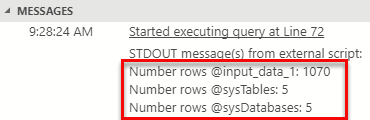It all works! Oh, it just so happens that I am on an almost new SQL Server instance, and that is why the number of rows in `sys.tables` and `sys.databases` is the same.

If R is your “poison of choice”, then we can, for example, use the `jsonlite` package. You can read more about it here. What we use from `jsonlite` is the `fromJSON` function:

 `````` 1 2 3 4 5 6 7 8 9 10 11 12 13 14 15 16 17 18 19 `````` ``````DECLARE @sysTabs nvarchar(max) = (SELECT * FROM sys.tables FOR JSON AUTO); DECLARE @sysDbs nvarchar(max) = (SELECT * FROM sys.databases FOR JSON AUTO); EXEC sp_execute_external_script @language = N'R' , @script = N' require(jsonlite) library(jsonlite) df <- InputDataSet sysTab <- as.data.frame(fromJSON(sysTables)) sysDb <- as.data.frame(fromJSON(sysDatabases)) cat(paste("Number rows @input_data_1: ", nrow(df))) cat(paste("\nNumber rows @sysTables: ", nrow(sysTab))) cat(paste("\nNumber rows @sysDatabases: ", nrow(sysDb)))' , @input_data_1 = N'SELECT * FROM sys.columns' , @params = N'@sysTables nvarchar(max), @sysDatabases nvarchar(max)' , @sysTables = @sysTabs , @sysDatabases = @sysDbs; ``````

Code Snippet 8: Parse JSON in R

The code in Code Snippet 8, looks very similar to what is in Code Snippet 7, and in Code Snippet 8 we:

• Load the `jsonlite` package.
• Assign the `InputDataSet` parameter to the `df` variable as before.
• Parse the JSON into data frames using `fromJSON`.

We have now seen how we, both in Python as well as R, can use JSON to push multiple datasets into external scripts, and - as I mentioned above - JSON is one way of doing it. Now onto the next.

#### Binary Serialization

When we use the binary serialization method of pushing multiple datasets, we use R and Python’s built-in functionality for serialization and deserialization. In Python, it is with the help of the `pickle` module, and in R the `serialize` and `unserialize` methods.

Initially, binary serialization looks somewhat more complicated than JSON (and it might be), especially since the deserialization happens against a binary parameter serialized with R or Python. In other words, we need to make a roundtrip to R/Python to get the binary representation of the data, so having a helper procedure to do this sounds like a good idea to me:

 `````` 1 2 3 4 5 6 7 8 9 10 11 12 `````` ``````CREATE PROCEDURE dbo.pr_SerializeHelperR @query nvarchar(max) , @serializedResult varbinary(max) OUT AS EXEC sp_execute_external_script @language = N'R' , @script = N' serRes <- serialize(InputDataSet, NULL)' , @input_data_1 = @query , @params = N'@serRes varbinary(max) OUT' , @serRes = @serializedResult OUT; ``````

Code Snippet 9: R Serialization Helper

In Code Snippet 9 we see an R serialization helper procedure:

• It takes a query as an input parameter (`query`).
• It has an output parameter which is the serialized result set.

The body of the procedure is a call to `sp_execute_external_script`, where the `@query` parameter acts as `@input_data_1`, and we have a defined output parameter `@serRes`. In the script, we call the R `serialize` function on the pushed in dataset, and assigns it to the output parameter. The flow:

• We pass in a query statement.
• During the call to `sp_execute_external_script` the query is run, and the resulting data set passed into the external script.
• The external script serializes the dataset and passes it back out as an output parameter.

The equivalent Python serialization helper looks like so:

 `````` 1 2 3 4 5 6 7 8 9 10 11 12 13 `````` ``````CREATE PROCEDURE dbo.pr_SerializeHelperPy @query nvarchar(max) , @serializedResult varbinary(max) OUT AS EXEC sp_execute_external_script @language = N'Python' , @script = N' import pickle as p serRes = p.dumps(InputDataSet)' , @input_data_1 = @query , @params = N'@serRes varbinary(max) OUT' , @serRes = @serializedResult OUT; ``````

Code Snippet 10: Python Serialization Helper

We see in Code Snippet 10 how we bring in the `pickle` module, and then serialize the dataset with the `dumps` function.

The equivalent of Code Snippet 7 using the Python serialization helper looks like so:

 `````` 1 2 3 4 5 6 7 8 9 10 11 12 13 14 15 16 17 18 19 20 21 22 23 24 25 26 27 28 29 30 31 32 33 34 35 36 37 38 `````` ``````-- declare the variables for queries as well -- as serialized binary representation DECLARE @sysTabs nvarchar(max) = 'SELECT name, object_id, schema_id FROM sys.tables'; DECLARE @sysDbs nvarchar(max) = 'SELECT name, database_id, source_database_id FROM sys.databases'; DECLARE @sysTabsBin varbinary(max); DECLARE @sysDbsBin varbinary(max); -- get the serialized result of @sysTabs EXEC dbo.pr_SerializeHelperPy @query = @sysTabs , @serializedResult = @sysTabsBin OUT -- get the serialized result of @sysDbs EXEC dbo.pr_SerializeHelperPy @query = @sysDbs , @serializedResult = @sysDbsBin OUT -- do the "real" stuff EXEC sp_execute_external_script @language = N'Python' , @script = N' import pandas as pd import pickle as p df = InputDataSet # this deserializes the sys.tables result sysTab = p.loads(sysTables) # this deserializes the sys.databases result sysDb = p.loads(sysDatabases) print("Number rows @input_data_1: ", len(df.index)) print("Number rows @sysTables: ", len(sysTab.index)) print("Number rows @sysDatabases: ", len(sysDb.index))' , @input_data_1 = N'SELECT * FROM sys.columns' , @params = N'@sysTables varbinary(max), @sysDatabases varbinary(max)' , @sysTables = @sysTabsBin , @sysDatabases = @sysDbsBin; ``````

Code Snippet 11: Implementation of Python Serialization

In Code Snippet 11 we see how we:

• Declare the variables for the queries as well as the serialized results of the queries.
• Execute the helper procedure for the two queries we want the results serialized for.
• Have defined two `varbinary(max)` parameters in the `@params` parameter of `sp_execute_external_script`.
• Assign the serialized values to those two parameters.
• Execute `sp_execute_external_script` and send in the two serialized results as well as `@input_data_1`.
• In `sp_execute_external_script` we deserialize the results using `pickle.loads`, or rather `p.loads` where `p` is the alias for `pickle`.

The code for an R implementation looks almost the same except that we call the R `unserialize` function instead of `pickle.loads`, as per the code below:

 `````` 1 2 3 4 5 6 7 8 9 10 11 12 13 14 15 16 17 18 `````` ``````-- declare the variables for the querie as well -- as serialized binary representation DECLARE @sysTabs nvarchar(max) = 'SELECT name, object_id, schema_id FROM sys.tables'; DECLARE @sysTabsBin varbinary(max); -- get the serialized result of @sysTabs EXEC dbo.pr_SerializeHelperR @query = @sysTabs , @serializedResult = @sysTabsBin OUT -- do the "real" stuff EXEC sp_execute_external_script @language = N'R' , @script = N' sysTab = unserialize(sysTables) cat(paste("Number rows @sysTables: ", nrow(sysTab)))' , @params = N'@sysTables varbinary(max)' , @sysTables = @sysTabsBin; ``````

Code Snippet 12: Implementation of R Serialization

We see in Code Snippet 12 how we push in one resultset, and we do not use `@input_data_1`. Instead, we serialize the resultset from the query with the R helper procedure and then deserialize with the `unserialize` function.

## Performance

So, we have now seen two ways of pushing in datasets to an external script: JSON and Binary Serialization. Which should you choose - I mean, JSON seems a lot easier? The answer comes down to performance.

To look at performance let us create a database, a table and some data:

 `````` 1 2 3 4 5 6 7 8 9 10 11 12 13 14 15 16 17 18 19 20 21 22 23 24 25 26 27 28 29 30 31 32 33 34 35 36 `````` ``````DROP DATABASE IF EXISTS MultiDataSetDB; GO CREATE DATABASE MultiDataSetDB; GO USE MultiDataSetDB GO SET NOCOUNT ON; GO DROP TABLE IF EXISTS dbo.tb_Rand1M ; CREATE TABLE dbo.tb_Rand1M ( RowID bigint identity, y int NOT NULL, rand1 int NOT NULL, rand2 int NOT NULL, rand3 int NOT NULL, rand4 int NOT NULL, rand5 int NOT NULL, CONSTRAINT [pk_Rand1M] PRIMARY KEY (RowID) ) GO INSERT INTO dbo.tb_Rand1M(y, rand1, rand2, rand3, rand4, rand5) SELECT TOP(1000000) CAST(ABS(CHECKSUM(NEWID())) % 14 AS INT) , CAST(ABS(CHECKSUM(NEWID())) % 20 AS INT) , CAST(ABS(CHECKSUM(NEWID())) % 25 AS INT) , CAST(ABS(CHECKSUM(NEWID())) % 14 AS INT) , CAST(ABS(CHECKSUM(NEWID())) % 50 AS INT) , CAST(ABS(CHECKSUM(NEWID())) % 100 AS INT) FROM sys.objects o1 CROSS JOIN sys.objects o2 CROSS JOIN sys.objects o3 CROSS JOIN sys.objects o4; GO ``````

Code Snippet 13: Database with Table and Data

We see above, in Code Snippet 13, how we create a database, with a table and we load one million records into the table. The data is entirely random and fairly useless, but it serves its purposes.

NOTE: If you code along and use the code above, please also create `dbo.pr_SerializeHelperPy`, which you see in Code Snippet 10, in the `MultiDataSetDB` database.

Having created the database and the objects, let us look at the code we use to compare performance. The code below is for JSON:

 `````` 1 2 3 4 5 6 7 8 9 10 11 12 13 14 15 `````` ``````--this is for JSON DECLARE @start datetime2 = SYSUTCDATETIME(); DECLARE @rand1M nvarchar(max) = (SELECT TOP(100) * FROM dbo.tb_Rand1M FOR JSON AUTO); EXEC sp_execute_external_script @language = N'Python' , @script = N' import pandas as pd df = pd.read_json(randTab) print("Number rows @randTab: ", len(df.index))' , @params = N'@randTab nvarchar(max)' , @randTab = @rand1M; SELECT DATEDIFF(ms, @start, SYSUTCDATETIME()) AS JSONTime; GO ``````

Code Snippet 14: JSON Performance Code

And here is the binary serialization code:

 `````` 1 2 3 4 5 6 7 8 9 10 11 12 13 14 15 16 17 18 19 20 21 22 23 24 25 `````` ``````--this is binary -- declare the variables for queries as well --as serialized binary representation DECLARE @start datetime2 = SYSUTCDATETIME(); DECLARE @rand1M nvarchar(max) = 'SELECT TOP(100) * FROM dbo.tb_Rand1M'; DECLARE @rand1MBin varbinary(max); -- get the serialized result of @sysTabs EXEC dbo.pr_SerializeHelperPy @query = @rand1M , @serializedResult = @rand1MBin OUT -- do the "real" stuff EXEC sp_execute_external_script @language = N'Python' , @script = N' import pandas as pd import pickle as p sysTab = p.loads(randTab) print("Number rows @sysTables: ", len(sysTab.index))' , @params = N'@randTab varbinary(max)' , @randTab = @rand1MBin SELECT DATEDIFF(ms, @start, SYSUTCDATETIME()) AS BinaryTime; GO ``````

Code Snippet 15: Binary Serialization Performance Code

The code in snippets 14, and 15 is a variant of what we have seen so far. In these two snippets, we only push in one table, and we look at the time it takes to do it. We see how we `SELECT` from `dbo.tb_Rand1M`, and initially, we do a `TOP(100)`.

When I highlight both code snippets and run them a couple of times to not incur “startup” costs the results are: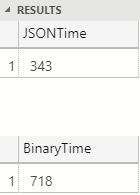Figure 4: Performance 100 Rows

That’s quite interesting; we see in Figure 4 how JSON serialization is around twice as fast as binary serialization. Ok, what if we did it on 1000 rows? With 1000 rows JSON is still about twice as fast. However, when we tun it against the full table (one million rows), the results are different: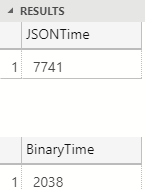Figure 5: Performance a Million Rows

In Figure 5 we see how with a million rows, the binary serialization is about 3.5 times faster than JSON. There are a couple of reasons why binary serialization performs better that JSON with larger datasets:

• Binary serialization is more compact, less data to transfer.
• By using the `@input_data_1` parameter to push in the data to the serialization we get a better performing transport. Read more about it in my post: Microsoft SQL Server R Services - Internals XIV.

So, for very small datasets, use the JSON method, but for larger datasets, the binary serialization is always preferred. Another thing to keep in mind is if you use both `@input_data_1` as well as pushing in serialized data (like in all our code snippets where we used three tables), try to use `@input_data_1` for the biggest dataset. That way you get the better performing transport and also, potentially, parallel execution of the query.

## Summary

We have now seen how we can push in multiple datasets to an external script, without having to connect from the script back to the database. We can use two methods:

• JSON.
• Binary serialization.

When we use JSON we utilize SQL Server’s JSON capabilities to execute a query and receive the result as JSON formatted text: `SELECT ... FROM ... FOR JSON AUTO`. In the external script we then deserialize the JSON using:

• In R the `fromJSON` function in the `jsonlite` package.
• In Python the `read_json` function in the `pandas` package.

When we do binary serialization we use R/Python’s capabilities to both serialize as well as deserialize data. This means we need to do a roundtrip to R/Python to serialize the data. The tools we use:

• In R we call `serialize` and `unserialize`.
• In Python we use functions from the `pickle` package. To serialize we call `dumps` and to deserialize we use `loads`.

What method to use (JSON or binary serialization) comes down to, in my mind, performance. For very small datasets JSON is faster, but as soon as the dataset gets bigger binary serialization outperforms JSON by order of magnitude.

## ~ Finally

If you have comments, questions etc., please comment on this post or ping me.